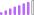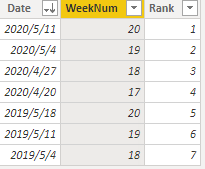cancel
Showing results for
Did you mean:Post Partisan

## 4 weeks average based on weekrank

Hi,

I would like to calculate the 4 weeks average based on weekrank.
example:
Weekno     rank     value
20              1           10

19               2           15

18               3           10

17               4            9

20              5             5

Weekno and rank comes from the datetable and value is calculated measure.
My desired outcome for weekno 20  4weeks average= 10+15+10+9 = 11 etc....

I tried the following the measure but it does not show me the average:
4 weeks average = var _ranktable = calculatedtable(values(rank);filter(allselected(rank);rank<=max(rank)+3 && rank>=max(rank)))
return
averagex =(_ranktable, value)

Any suggestions?

1 ACCEPTED SOLUTIONCommunity Support

Hi @Coan7

I build two table to have a test.

Date Table:

Weeknom and Rank columns are calculated columns.

``Weeknom = WEEKNUM('Date'[Date],2)``
``Rank = RANKX('Date','Date'[Date],,DESC,Dense)``

Result:Value Table:Build Value measure in Date table:

``Value = CALCULATE(SUM('Value'[Value]),FILTER('Value','Value'[Date]=MAX('Date'[Date])))``

Then I build a measure to achieve your goal.

``````4 weeks average =

VAR _Totalvalue= SUMX (

FILTER (

ALL ( 'Date' ),

'Date'[Rank]

<= MAX ( 'Date'[Rank] ) + 3

&& 'Date'[Rank] >= MAX ( 'Date'[Rank] )

),

[Value]

)

return

IF (

MAXX ( ALL ( 'Date' ), 'Date'[Rank] ) - SUM ( 'Date'[Rank] ) >= 3,

DIVIDE(_Totalvalue,4),

BLANK()

)``````

Result:Best Regards,

Rico Zhou

If this post helps, then please consider Accept it as the solution to help the other members find it more quickly.

5 REPLIES 5Community Support

Hi @Coan7

I build two table to have a test.

Date Table:

Weeknom and Rank columns are calculated columns.

``Weeknom = WEEKNUM('Date'[Date],2)``
``Rank = RANKX('Date','Date'[Date],,DESC,Dense)``

Result:Value Table:Build Value measure in Date table:

``Value = CALCULATE(SUM('Value'[Value]),FILTER('Value','Value'[Date]=MAX('Date'[Date])))``

Then I build a measure to achieve your goal.

``````4 weeks average =

VAR _Totalvalue= SUMX (

FILTER (

ALL ( 'Date' ),

'Date'[Rank]

<= MAX ( 'Date'[Rank] ) + 3

&& 'Date'[Rank] >= MAX ( 'Date'[Rank] )

),

[Value]

)

return

IF (

MAXX ( ALL ( 'Date' ), 'Date'[Rank] ) - SUM ( 'Date'[Rank] ) >= 3,

DIVIDE(_Totalvalue,4),

BLANK()

)``````

Result:Best Regards,

Rico Zhou

If this post helps, then please consider Accept it as the solution to help the other members find it more quickly.Helper III

Hi @v-rzhou-msft this is extremly helpful - but I would like to do it for the last 4 weeks excluding the latest week - so here in this example, I want to average rank 2 to 5 instead of 1 to 4. How can I adjust your formula to do that? Thanks so much!Super UserPost Partisan
Tnx for your reply, but this does not seem to work for this case.Super User

@Coan7 , Try like

Last 4 Week = CALCULATE(Average(Table[value]),FILTER(all('Table'),'Table'[Rank]>=min('Table'[Rank])-4
&& 'Table'[Rank]<=max('Table'[Rank])))

Last 4 Week = CALCULATE(Average(Table[value]),FILTER(allselected('Table'),'Table'[Rank]>=min('Table'[Rank])-4
&& 'Table'[Rank]<=max('Table'[Rank])))

But prefer to have a week table and week and rank there

Like

Last 4 Week = CALCULATE(Average(Table[value]),FILTER(all('WEEK'),'WEEK'[Rank]>=min('WEEK'[Rank])-4
&& 'WEEK'[Rank]<=max('WEEK'[Rank])))

Last 4 Week = CALCULATE(Average(Table[value]),FILTER(allselected('WEEK'),'WEEK'[Rank]>=min('WEEK'[Rank])-4
&& 'WEEK'[Rank]<=max('WEEK'[Rank])))